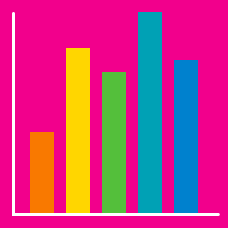Probability

# Data Presentation - Pie Charts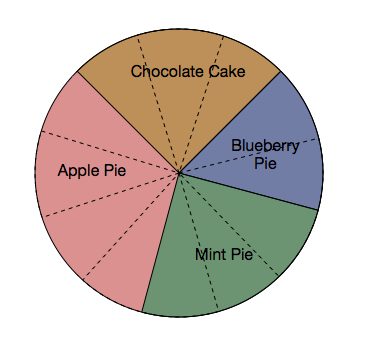The above pie chart (where each dotted slice represents a fixed number of pies) shows the number of desserts that are sold in a diner. If $12$ pies were sold, how many apple pies were sold?The above pie chart (where each dotted slice represents the same number of desserts) shows the distribution of desserts that are sold in a diner. If $26$ blueberry pies were sold, how many chocolate cakes were sold?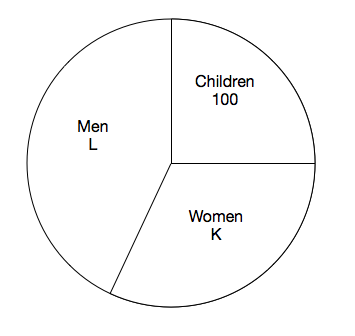At a country fair, $\frac{1}{4}$ of the attendees were children. How many people attended the fair?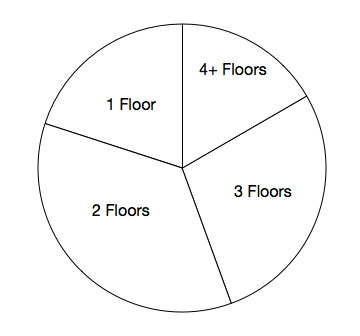The above pie chart shows the distribution of the number of floors in homes of a certain city. What is the mode of this distribution?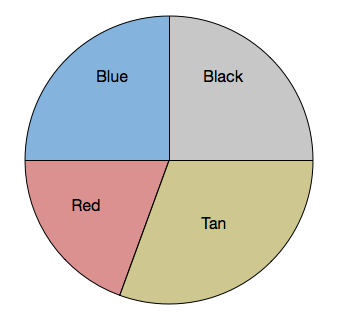The above pie chart represents the distribution of socks that are in a drawer. What other color has an equal number of socks as blue?

×